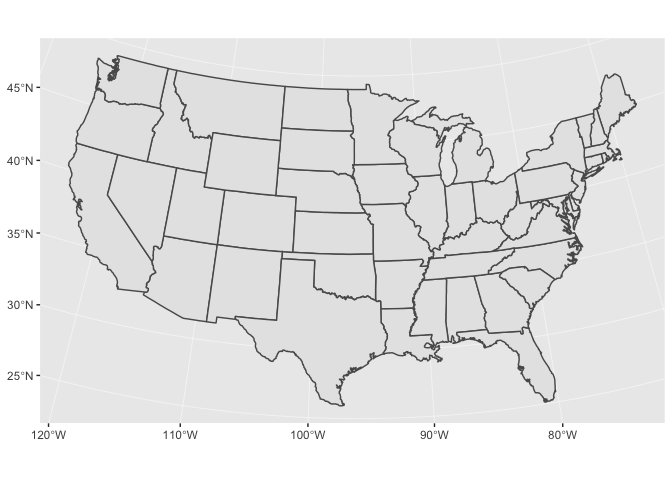# Spatial visualization [visualize]

(Builds on: Spatial basics)

## Setup

We’ll start by reading in a couple of sample data sets, as well as loading the sf package.

``````library(tidyverse)
library(sf)

nc_1974 <-
select(FIPS, NAME, births = BIR74, sids = SID74,  geometry) %>%
rename_all(str_to_lower)

states <- st_as_sf(maps::map("state", plot = FALSE, fill = TRUE))
``````

In Spatial basics, you learned how to plot spatial data using the `plot()` function. Now, we’ll show you how to visualize spatial data using `geom_sf()`. The advantage of `geom_sf()` is that, as part of ggplot2, you can layer geoms, use scales functions to change colors, tweak your legends, alter the theme, etc.

## `geom_sf()`

`nc_1974` contains data on deaths from Sudden Infant Death Syndrome (SIDS) in 1974 for counties in North Carolina.

``````nc_1974
#> Simple feature collection with 100 features and 4 fields
#> geometry type:  MULTIPOLYGON
#> dimension:      XY
#> bbox:           xmin: -84.32385 ymin: 33.88199 xmax: -75.45698 ymax: 36.58965
#> epsg (SRID):    4267
#> # A tibble: 100 x 5
#>    fips  name     births  sids                                     geometry
#>    <chr> <chr>     <dbl> <dbl>                           <MULTIPOLYGON [°]>
#>  1 37009 Ashe       1091     1 (((-81.47276 36.23436, -81.54084 36.27251, …
#>  2 37005 Allegha…    487     0 (((-81.23989 36.36536, -81.24069 36.37942, …
#>  3 37171 Surry      3188     5 (((-80.45634 36.24256, -80.47639 36.25473, …
#>  4 37053 Curritu…    508     1 (((-76.00897 36.3196, -76.01735 36.33773, -…
#>  5 37131 Northam…   1421     9 (((-77.21767 36.24098, -77.23461 36.2146, -…
#>  6 37091 Hertford   1452     7 (((-76.74506 36.23392, -76.98069 36.23024, …
#>  7 37029 Camden      286     0 (((-76.00897 36.3196, -75.95718 36.19377, -…
#>  8 37073 Gates       420     0 (((-76.56251 36.34057, -76.60424 36.31498, …
#>  9 37185 Warren      968     4 (((-78.30876 36.26004, -78.28293 36.29188, …
#> 10 37169 Stokes     1612     1 (((-80.02567 36.25023, -80.45301 36.25709, …
#> # … with 90 more rows
``````

First, let’s just plot the county boundaries. `geom_sf()` works like the other geoms, except that you don’t need to specify an aesthetic mapping. By default, `geom_sf()` will plot your `geometry` column.

``````nc_1974 %>%
ggplot() +
geom_sf()
``````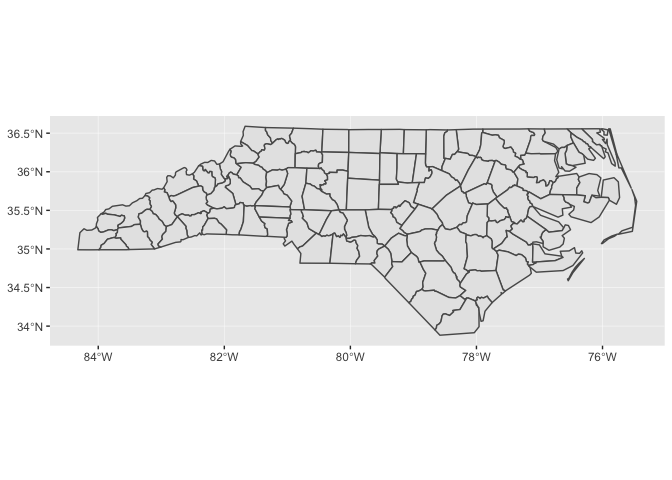Notice that ggplot2 takes care of setting the aspect ratio correctly. If we just plot a single county in North Carolina, the default aspect ratio will be different.

``````nc_1974 %>%
filter(name == "Anson") %>%
ggplot() +
geom_sf()
``````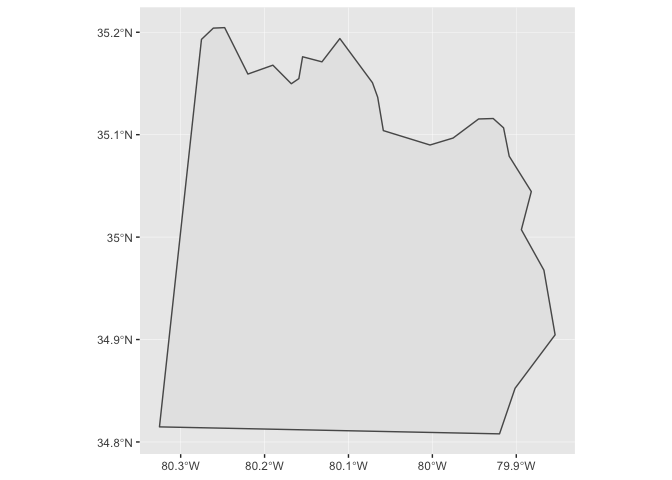Just like with other geoms, you can supply additional aesthetics. For polygons like the counties of North Carolina, `fill` is the most useful aesthetic. Let’s visualize the number of births by county.

``````nc_1974 %>%
ggplot(aes(fill = births)) +
geom_sf(color = "white", size = 0.2)
``````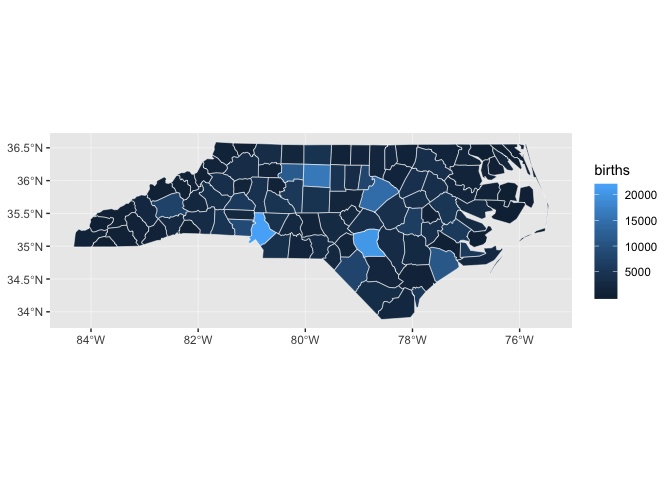Maps like this one, in which geographic areas are colored according to some variable, are called choropleths.

``````nc_1974 %>%
ggplot(aes(fill = births)) +
geom_sf(color = "white", size = 0.2)
``````Notice how it’s difficult to tell the difference between many of the counties, especially those with very low numbers of births.

Let’s look at the distribution of births.

``````nc_1974 %>%
ggplot(aes(births)) +
geom_histogram(binwidth = 500) +
scale_x_continuous(breaks = seq(0, 2e5, 2e3))
``````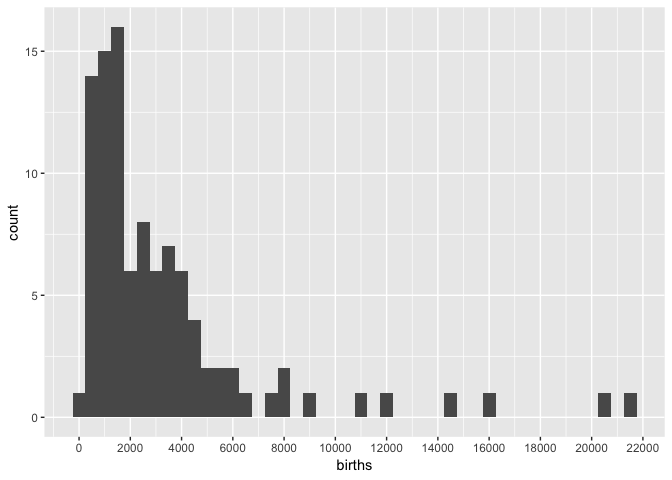Almost all of the counties fall between 0 and 8,000, but because there are some counties with births up to 22,000, the sequential color scale will linearly map colors between 0 and 22,000.

One way to deal with this problem is to cap `births`.

``````nc_1974 %>%
mutate(births = pmin(births, 1e4)) %>%
ggplot(aes(fill = births)) +
geom_sf()
``````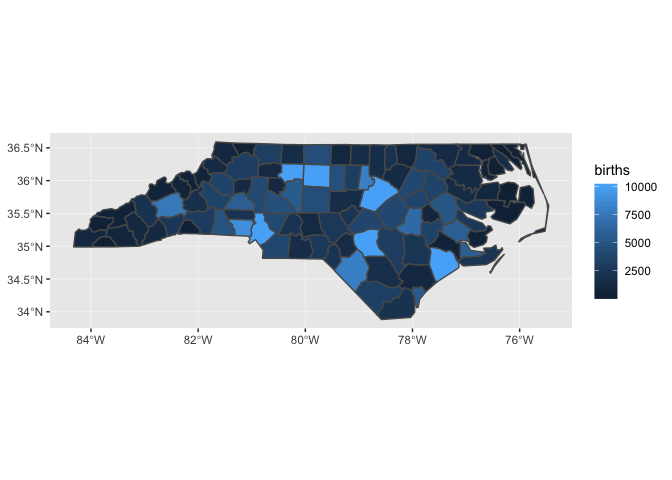## Layering

`states` and `nc_1974` each has a different coordinate reference system (CRS).

``````st_crs(states)
#> Coordinate Reference System:
#>   EPSG: 4326
#>   proj4string: "+proj=longlat +datum=WGS84 +no_defs"

st_crs(nc_1974)
#> Coordinate Reference System:
#>   EPSG: 4267
``````

When you include multiple geospatial layers, ggplot2 will ensure that they all have a common CRS so that it makes sense to overlay them. It will use the CRS of the first layer for all layers.

``````ggplot() +
geom_sf(data = states) +
geom_sf(data = nc_1974)
``````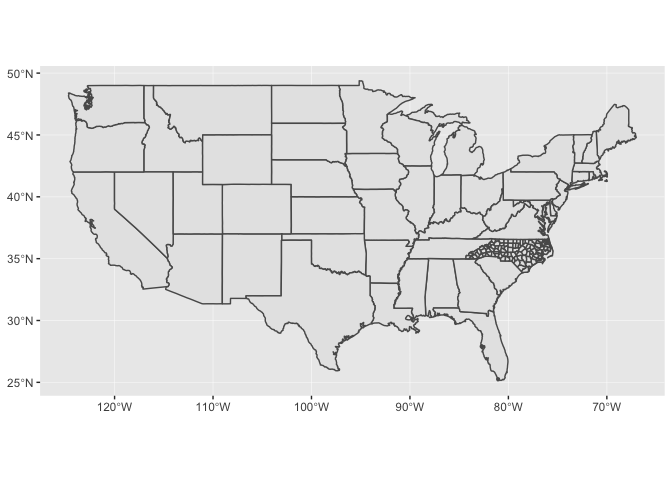In the above code, we didn’t pipe any data into `ggplot()` because each layer uses different data.

Because `geom_sf()` is part of ggplot2, you can combine `geom_sf()` with other geoms.

``````raleigh <-
tibble(
x = -78.6382,
y = 35.7796,
label = "Raleigh"
)

nc_1974 %>%
mutate(births = pmin(births, 1e4)) %>%
ggplot() +
geom_sf(aes(fill = births)) +
geom_point(aes(x, y), color = "red", data = raleigh) +
geom_label(aes(x, y, label = label), nudge_x = 0.8, data = raleigh) +
scale_fill_gradient(low = "#efedf5", high = "#756bb1")
``````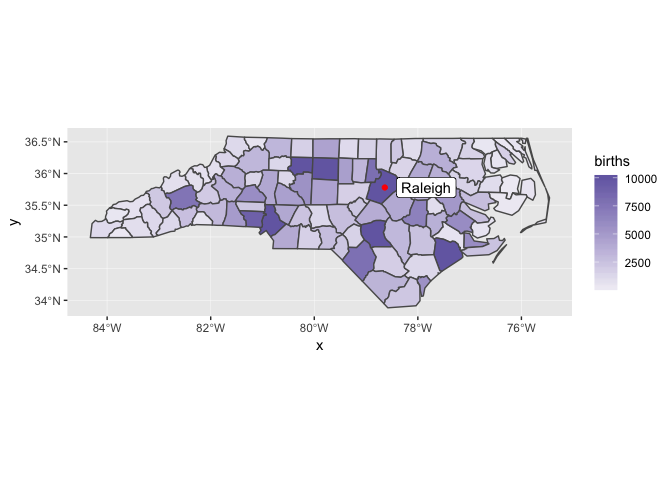In this case, the `x` and `y` positions of the Raleigh dot are assumed be in the same CRS as the sf object.

You can find this lighter color scale, and many others, at the ColorBrewer website.

## `coord_sf()`

You’ll need to use `coord_sf()` for two reasons:

• You want to zoom into a specified region of the plot.

The following plot shows the SIDS rate by county.

``````nc_1974 <-
nc_1974 %>%
mutate(sids_rate = sids / births)

nc_1974 %>%
ggplot(aes(fill = sids_rate)) +
geom_sf()
``````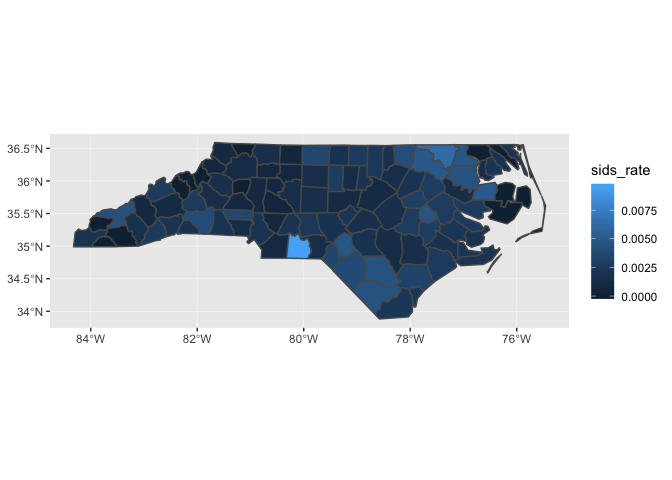Let’s zoom in on the county with the highest rate (and also change the color scale).

``````nc_1974 %>%
ggplot(aes(fill = sids_rate)) +
geom_sf() +
scale_fill_gradient(low = "#efedf5", high = "#756bb1") +
coord_sf(xlim = c(-80.5, -79), ylim = c(34.8, 36))
``````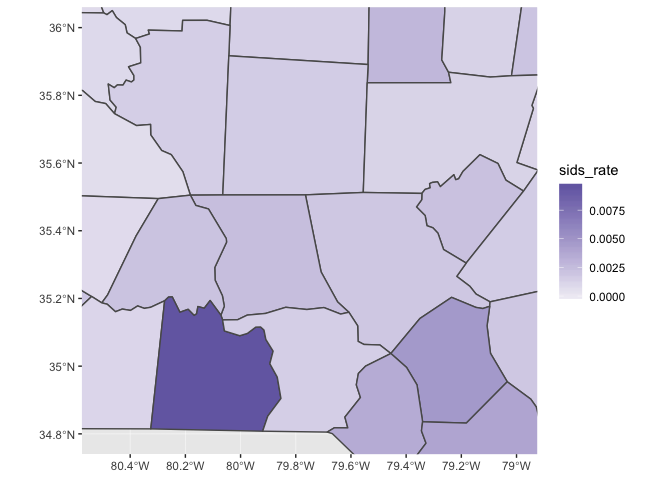• You can also use `coord_sf()` to override the projection in the data. If you don’t specify the `crs` argument to `coord_sf()`, if defaults to the CRS given in the data.

The CRS for the `states` data is

``````states %>%
st_crs()
#> Coordinate Reference System:
#>   EPSG: 4326
#>   proj4string: "+proj=longlat +datum=WGS84 +no_defs"
``````

which produces a map that looks like this:

``````states %>%
ggplot() +
geom_sf()
``````If you’re visualizing the entire US, it’s better to use the US Albers projection. Albers is an equal-area projection, which means it accurately reflects the areas of geographic regions. Below is the proj.4 string for the US Albers projection.

``````US_ALBERS <- "+proj=aea +lat_1=29.5 +lat_2=45.5 +lat_0=37.5 +lon_0=-96 +x_0=0 +y_0=0 +datum=WGS84 +no_defs"

states %>%
ggplot() +
geom_sf() +
coord_sf(crs = US_ALBERS)
``````